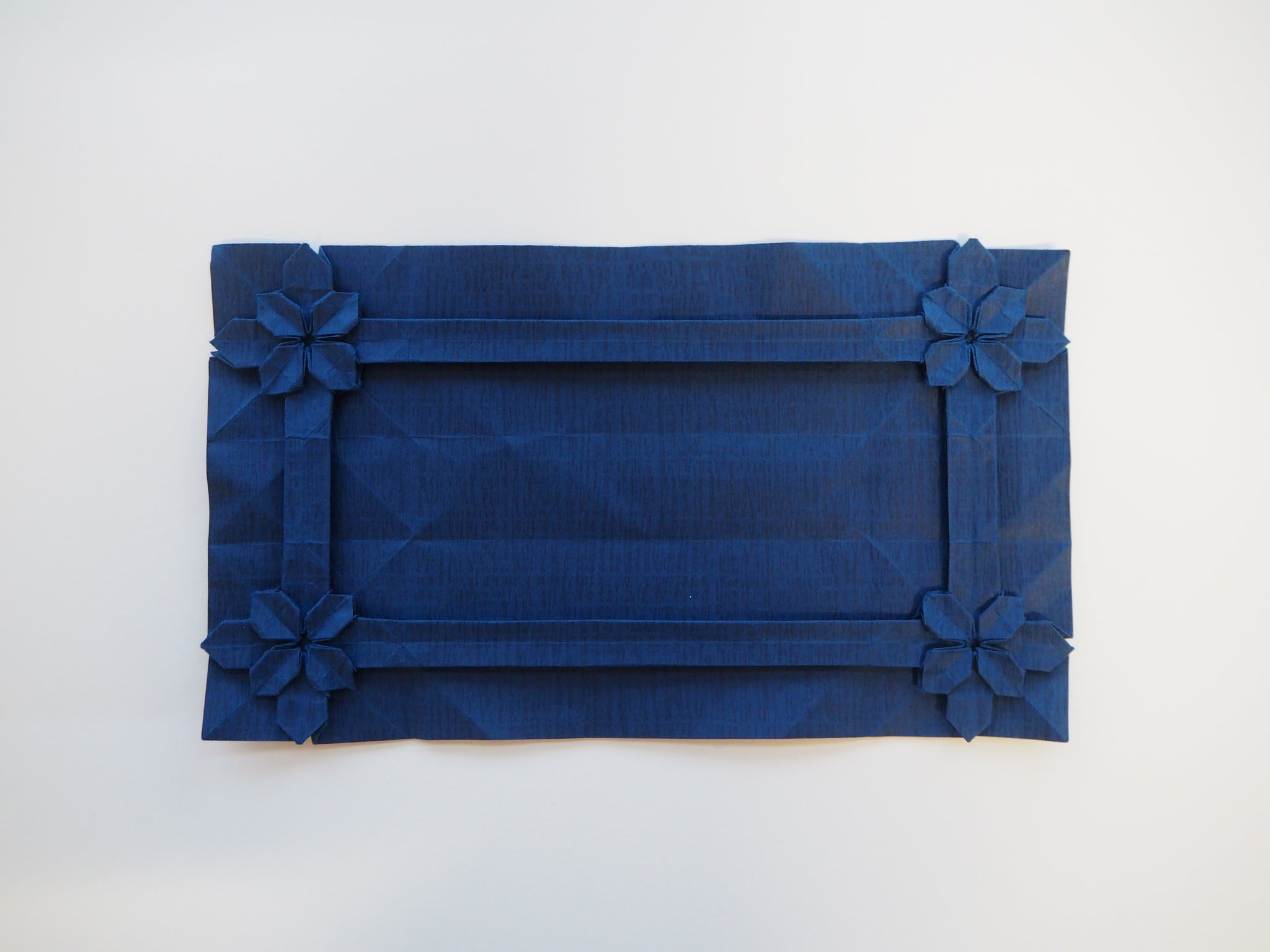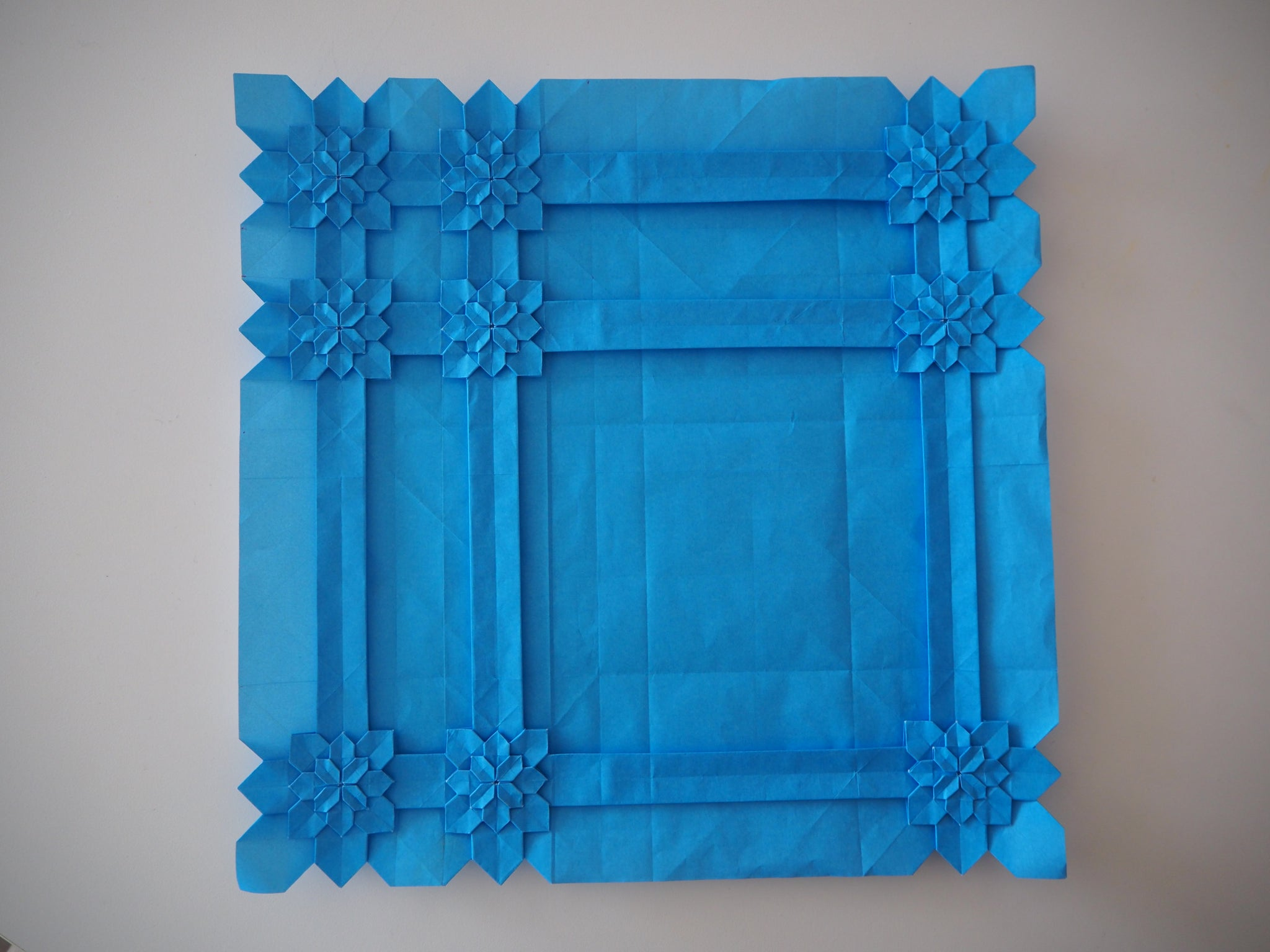# ORIGAMI HYDRANGEA TESSELLATIONS

Origami Hydrangea tiling.

Hydrangea tessellation is designed by Shuzo Fujimoto.

On our previous blog we talked about the basic hydrangea tessellation. To move to another level. We are going to show how to do tiling by adjusting number of pleats.

A standard hydrangea tile needs 8x8 equal squares.Multiple tiles on one paper :If we lower the density by one column of squares,  we can use the equation:

8+ 7(n-1)

"n" is the number of tiles you want to do in one column or one row.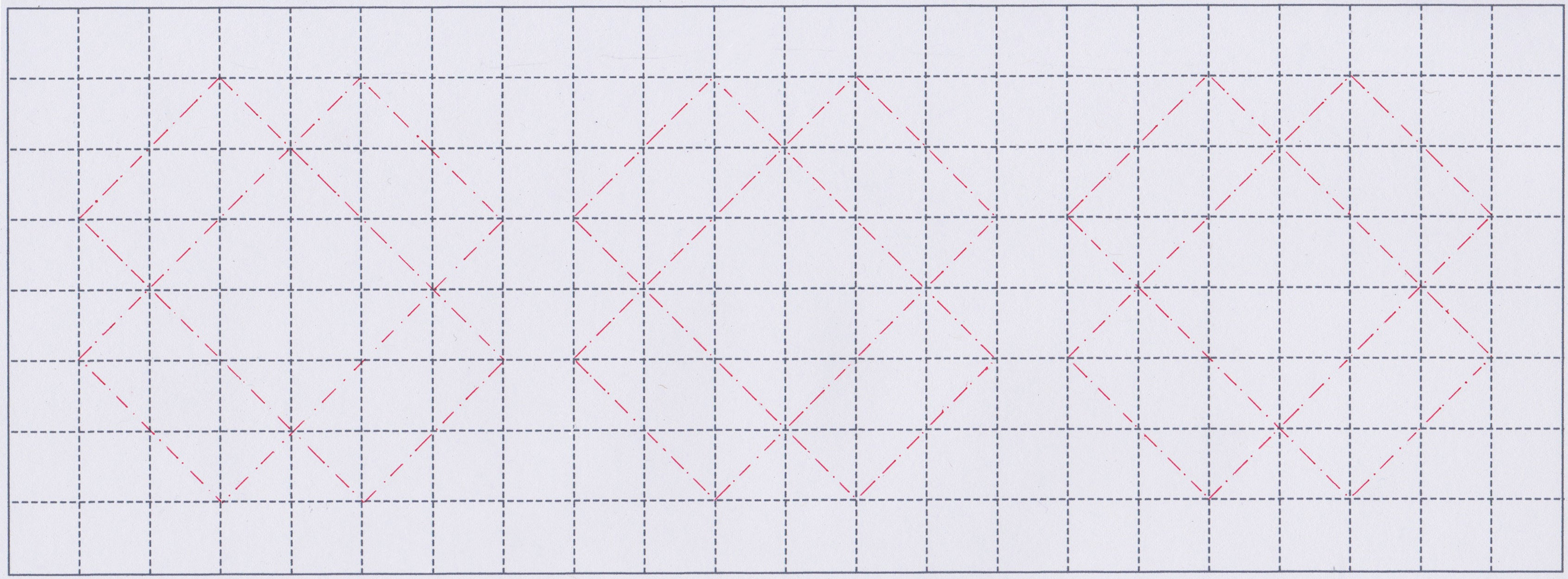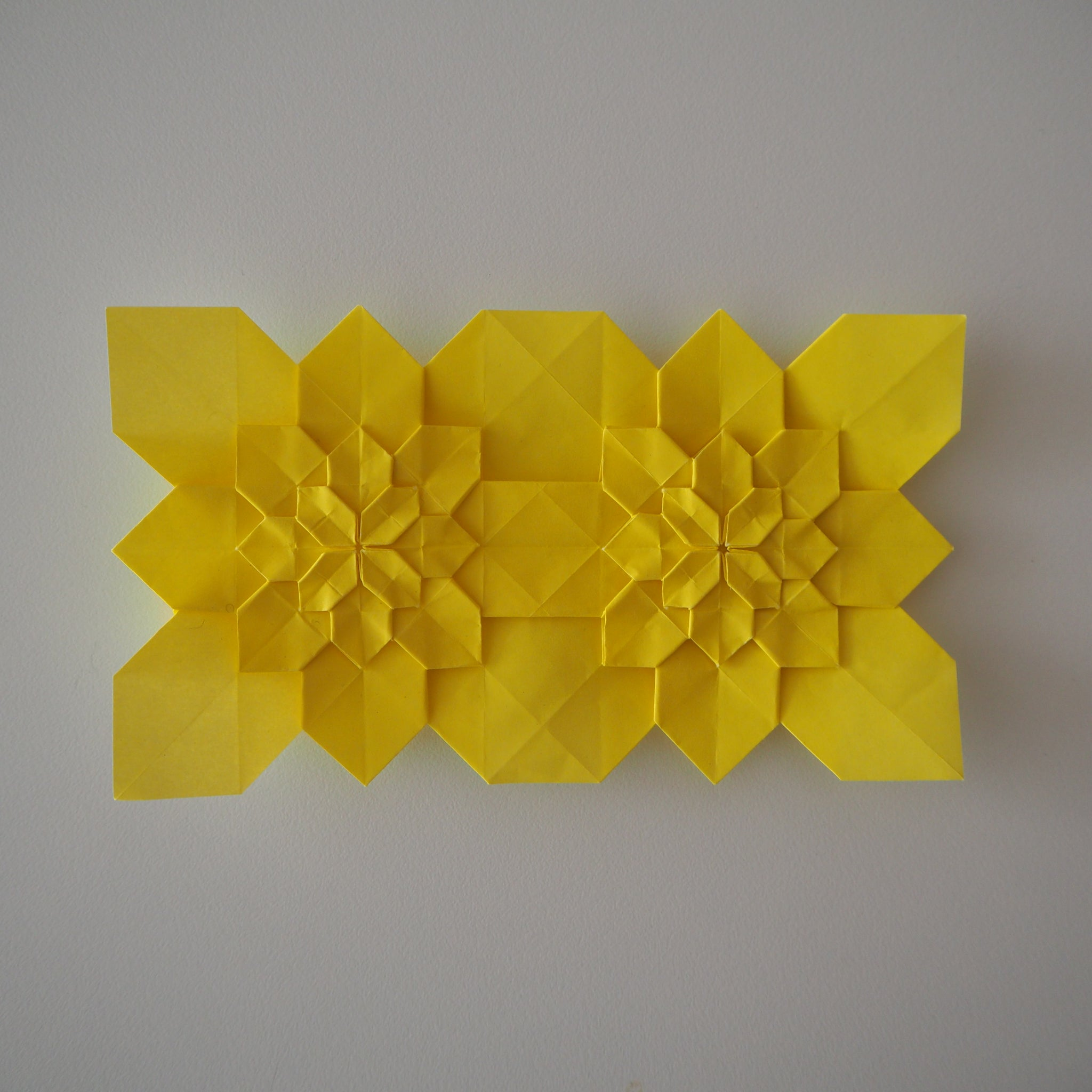If we reduce the density by sharing two column of squares between two tiles, use the following equation to calculate how many squares you need in one column or one row.

8 +6 (n-1)

For example: I would like to do two tiles in a row. So it is 8+6(2-1) = 14 ; The result is 14 squares per row and still 8 square in a column.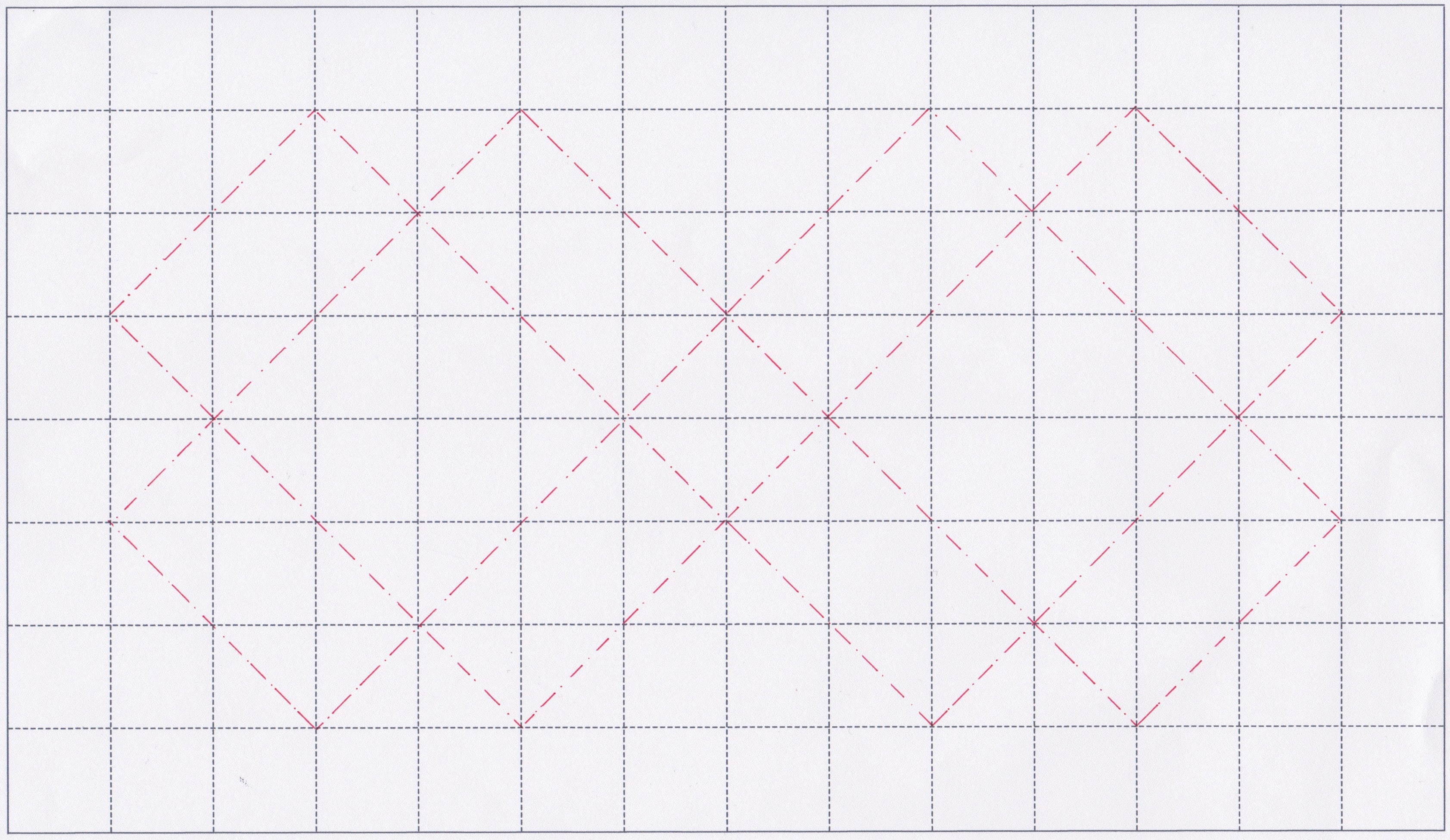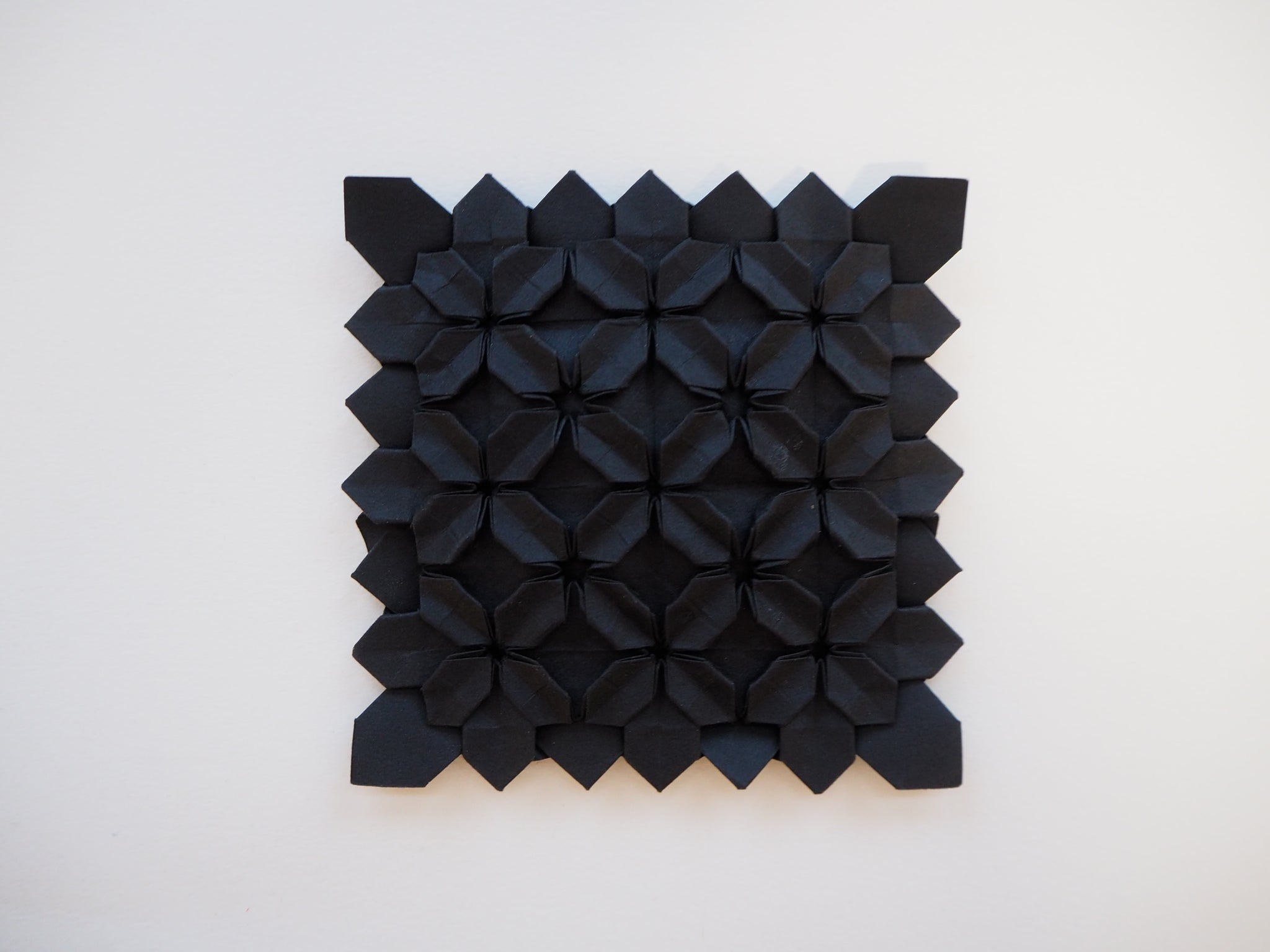Four tiles in a row will be 8+6(4-1)=26, that gives 26 squares in a row. Same way to increase the number of tile on both directions.

There is no limits. Try your own tilings.